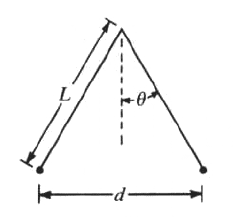GR 8677927796770177 | # Login | Register

GR9277 #83
Problem
 GREPhysics.NET Official Solution Alternate Solutions
\prob{83}Two pith balls of equal mass M and equal charge q are suspended from the same point on long massless threads of length L as shown in the figure above. If k is the Coulomb's law constant, then for small values of , the distance d between the charged pith balls at equilibrium is

Electromagnetism}Forces

Sum of the forces for one of the mass in the x (horizontal) and y (vertical) directions gives,

For small angles, . From the geometry, one can deduce that .

Thus, the x equation yields (since from the y equation for small angles). This is choice (A).Alternate Solutions
 cjohnson4152013-08-19 01:40:11 Here's an alternative solution that skips the trig altogether: Due to small , we can consider each as a simple pendulum, a special case of the simple harmonic oscillator: Thus , which is the restoring force constant (), so: The rest is the same algebra, just a different/potentially quicker/potentially prettier way to arrive at the force balance.Reply to this commentneo553780082012-08-18 12:27:15 Dimensional analysis helps! Since is in N, will have units of Mg has units of N so will have units of This leaves only A and B. Knowing that solving for will give half of d, take the solution with an extra 2 in it! (A)Reply to this commentredmomatt2011-11-06 08:25:21 Heres another take without the trig functions... Since is small, we can remove all of the trig functions. Thus, we just need to equate the outward Coulomb force to the force of gravity along the horizontal . , since . Hence, , which is (B) .Reply to this commentAndresito2006-03-29 14:56:46 It is a bit faster to equate F(electric) = T*Sin(theta), m*g = T*Cos(theta) and divide them. Tan(theta) ~ thetaReply to this commentastropolo
2014-07-29 11:55:16
I have a quick question about the small angle approximations. Why can you approximate that Cos~1 while you use the geometry for Sin rather than saying sin~theta?
 JoshWasHere2014-08-20 08:20:22 When you expand cosine and sine, you see that the first two terms in the cosine and sine expansion are cos = 1 - and sin = - . Because of the small angle, we select only the first order terms, and drop all higher order terms (with the condition that < 5 degrees).cjohnson415
2013-08-19 01:40:11
Here's an alternative solution that skips the trig altogether:

Due to small , we can consider each as a simple pendulum, a special case of the simple harmonic oscillator:

Thus , which is the restoring force constant (), so:

The rest is the same algebra, just a different/potentially quicker/potentially prettier way to arrive at the force balance.greatspirits
2012-11-02 15:49:28
Energy conservation anyone? The change in gravitational potential energy should equate to potential between the charges. So... should equate to... hmmm well I usually prefer the energy method but the force way seems to be more effecient here.neo55378008
2012-08-18 12:27:15
Dimensional analysis helps!
Since is in N, will have units of
Mg has units of N
so will have units of
This leaves only A and B. Knowing that solving for will give half of d, take the solution with an extra 2 in it! (A)
 henryb2016-09-12 18:14:40 thx a lot. this is the simplest solutionredmomatt
2011-11-06 08:25:21
Heres another take without the trig functions...

Since is small, we can remove all of the trig functions. Thus, we just need to equate the outward Coulomb force to the force of gravity along the horizontal .

, since .

Hence,

, which is (B) .Dodobird
2010-11-12 11:57:31
tan(theta) = d/2L
tan(theta) = kq*q/d*d*MG

d/2L = kq*q/d*d*MGFortranMan
2008-10-03 14:02:49
Also note because theta is so small here, we can approximate the vector component of the gravity that's NOT inducing tension to be d/2.JAS
2007-11-01 09:20:45
It seems easier to equate torques around the pivot point, where the torque on the right mass due to the repulsive electric force isrnrn counterclockwisernrnand the torque on the right mass due to its weight is:rnrn clockwisernrn and rnrnFrom this, choice A follows.relain
2007-10-23 19:21:51
typo in the question, option D should be a square not a cube root. It's currently identical to option B.Andresito
2006-03-29 14:56:46
It is a bit faster to equate F(electric) = T*Sin(theta), m*g = T*Cos(theta) and divide them. Tan(theta) ~ thetatan(theta) = d/2L tan(theta) = kq*q/d*d*MG d/2L = kq*q/d*d*MG gives answer A.LaTeX syntax supported through dollar sign wrappers $, ex.,$\alpha^2_0$produces . type this... to get...$\int_0^\infty\partial\Rightarrow\ddot{x},\dot{x}\sqrt{z}\langle my \rangle\left( abacadabra \right)_{me}\vec{E}\frac{a}{b}\$